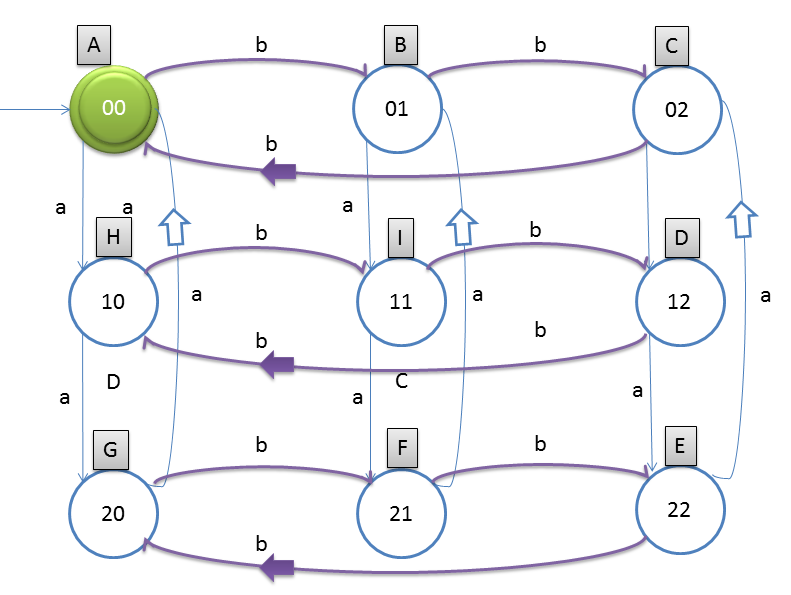# DFA String Examples

Design a DFA such a way that
Let string is w, Number of a(w) mod 3= 0 and Number of b(w) mod 3= 0. This means number of a should be divisible by 3 as well as number of b should also be divisible by 3.

If you see this question it is also just a bit variation of the above question.
The notations which we have used, we can choose which will be final state.Note
1. Alphabets in caps represent state name
2. Alphabets in small letters represent transition from one state to another
3. digits inside the states show number of a’s and b’s till that state.(divided by 3 off course)

### Testing

1. Take string ababab and traverse from left to right, here #a = 3 and #b = 3
2. from left first input is ‘a’ and start state ‘A’
3. so from state A we will go to state H
4. second input is ‘b’ and from H on b we will go to I
5. third input is ‘a’ and from I on a we will go state F
6. fourth input is ‘b’ and from F on b we will go to state E
7. fifth input is ‘a’ and from E on b we will go to state C
8. sixth input is ‘b’ and from C on b we will go to state A
After end of the string we are standing at state A, which is final state. So we say that string got accepted. Note: Take several examples and try out yourself.

#### Trick:

When such kind of question is asked in gate and they will just ask how many states are possible?
Answer: Suppose question is #a mod n = i and/or #b mod m = j then
Number of states = n * m